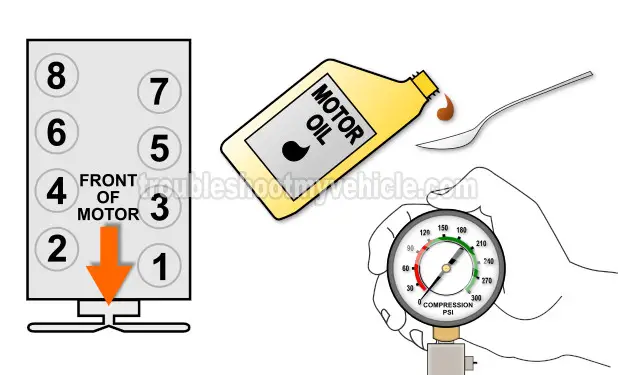# How To Do An Engine Compression Test (1993-1998 5.2L Jeep Grand Cherokee)

## Interpreting The Engine Compression Test Results

It's normal for the cylinder compression values to vary a bit, since the cylinders don't wear out at the exact same rate.

The more wear and tear and engine has, the more these values will vary. What will cause a misfire or rough idle condition is when the lowest cylinder compression value varies by more than 15% of the highest cylinder compression value.

The cool thing is that figuring out if the cylinder is 'dead' due to low compression is not difficult at all.

You can do this one of two ways: You can calculate this 15% difference with pen and paper or you can use my low compression calculator. You can find the low compression calculator here: Online Low Engine Compression Calculator (at: easyautodiagnostics.com).

If you want to manually calculate the 15% difference, here's what you'll need to do:

• STEP 1: Multiply the highest compression value by 0.15 (this is the decimal value of 15%).
• STEP 2: Round the result to the nearest one (for example: 25.6 would become 26).
• STEP 3: Subtract the result (the number that was rounded) from the highest compression value.
• ANSWER: The result of this subtraction is the lowest possible compression value any cylinder can have.

Now, let me give you a more specific example: Let's say that I got the following compression readings:

Cylinder Pressure
#1 165 PSI
#2   95 PSI
#3 155 PSI
#4 175 PSI
#5 160 PSI
#6 165 PSI
#7 160 PSI
#8 165 PSI

My next step is to do the following calculation:

• STEP 1:  175 x 0.15 = 26.25.
• STEP 2:  26.25 = 26 (rounded to nearest one).
• STEP 3:  175 - 26 = 149.
• ANSWER:  149 PSI. Any cylinder with this compression (or lower) value will misfire.

Since cylinder #2 is only producing 95 PSI, I can now conclude that it's 'dead' and causing a misfire.

To find out if the lowest compression value you got from your engine compression test is within a good range, you'll need to do the same calculation. Of course, you'll need to use the highest compression value you got and not the one in the example.

Once you've found the 'dead' cylinder, the next step is to find out what's causing the low compression value. For this step, go to: TEST 2: Wet Engine Compression Test.

## TEST 2: Wet Engine Compression TestGenerally, two problems cause a cylinder to have low or 0 PSI compression value. These are:

• The cylinder's valves (in the cylinder head) are worn or damaged.
• The cylinder's piston rings are worn.

The cool thing is that we can easily find out by doing a 'wet' compression test. This test simply involves adding a small quantity of engine oil to the cylinder with low or 0 PSI compression and retesting its compression.

If the compression value, of that 'dead' cylinder increases, then you can conclude that its piston rings are the cause of the low or 0 PSI compression problem.

If the compression value does not increase, then you can conclude that its cylinder head valves are the ones behind the low or 0 PSI compression problem.

These are the test steps:

1. 1

Add a tablespoon (or two) of engine oil in the cylinder you need to retest.

Use a funnel to make sure that the oil reaches the inside of the cylinder.

2. 2

Install the compression gauge on the cylinder and hand tighten it.

3. 3

Have your helper crank the engine till the needle stops climbing on the compression gauge.

4. 4

You'll see one of two results:

1.) The needle will climb higher than the previous compression number you recorded for this specific cylinder.

2.) The needle will not move at all or stay at the same number you recorded earlier.

5. 5

If you have another cylinder to test, repeat steps 1 thru' 4 on it now.

Let's take a look at what test results mean:

CASE 1: The compression value increased. This tells you that the low compression problem is due to worn piston compression rings.

CASE 2: The compression value DID NOT increase (in other words, it stayed the same). This result tells you that the low compression value registered in this cylinder (in the dry test) is due to worn/damaged cylinder head valves.

## More 5.2L Jeep Grand Cherokee Tutorials

You can find a complete list of 5.2L Jeep Grand Cherokee tutorials in this index:

Here's a small sample of the tutorials you'll find in the index:If this info saved the day, buy me a beer!Jeep Vehicles:

• Grand Cherokee 5.2L V8
• 1993,
1994,
1995,
1996,
1997,
1998